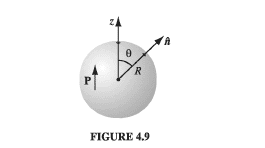# Bound charge question

Tony Hau
Homework Statement:
Find the electric field produced by a uniformly polarized sphere of radius R.
Relevant Equations:
Potential of a polarized object: ##V(\mathbf r) = \frac{1}{4 \pi \epsilon_o} \oint_{S} \frac{\sigma_b}{r}{da} + \frac{1}{4\pi \epsilon_o} \int_V \frac{\rho_b}{r} d{\tau} ##

Charged density of bound charge: ##\rho_b \equiv - \nabla \cdot \mathbf P##

Surface charge density of bound charge: ##\sigma_b \equiv \mathbf P \cdot \hat {\mathbf n} ##
This is an example of Griffith's book on bound charge, and the following is the solution to this example.

We choose the z-axis to conincide with the direction of polarization.

By $$\sigma_b \equiv \mathbf P \cdot \hat {\mathbf n}$$ and $$\rho_b \equiv - \nabla \cdot \mathbf P$$ we can see that ##\rho_b## is 0 and ##\sigma_b = Pcos\theta##.

The book then gives the solution to the potential without steps, which is: $$V(r,\theta) = \begin{cases} \frac{P}{3 \epsilon_o} rcos\theta & \text{for} \text{ }r \leq R \\ \frac{P}{3\epsilon_o}\frac{R^3}{r^2}cos\theta & \text{for } \text{ }r \geq R \end{cases}$$

This is a figure from the book:My attempt to the solution is like this:
$$V(\mathbf r) = \frac{1}{4 \pi \epsilon_o} \oint_{S} \frac{\sigma_b}{r}{da}$$, because ##\rho_b = 0##.

Then, ##\oint_{S} \frac{\sigma_b}{r}da= \int_0^ {\pi}\int_0^{2\pi} \frac{Pcos\theta}{R} R^{2}sin\theta d\theta d\phi##

I think the variable r is a constant because it is integrating over a surface; the r is fixed. However, it is not the case for the solution. Can anyone help?

Last edited:

Homework Helper
2022 Award
I don't think the integral formulation will necessarily help you; I believe it should be $$V(\mathbf{r}) = \int_S \frac{\sigma_b}{\|\mathbf{r} - \mathbf{r}'\|}\,dS'$$ where the integration is with respect to the primed variable.

I would instead note that since $\rho = 0$ the potential satisfies $$\nabla^2 V = 0$$ in $r < R$ and $r > R$.

I don't know enough EM to say what boundary condition it must satisfy at $r = R$ due to the charged sphere (presumably Griffiths will tell you), but I suspect that it will end up being a condition of the form $\frac{\partial V}{\partial r} = A\cos\theta$ for some constant $A$. That would suggest looking for solutions of the form $$V(r) = f(r) \cos \theta.$$

•Tony Hau
Tony Hau
I don't think the integral formulation will necessarily help you; I believe it should be $$V(\mathbf{r}) = \int_S \frac{\sigma_b}{\|\mathbf{r} - \mathbf{r}'\|}\,dS'$$ where the integration is with respect to the primed variable.

I would instead note that since $\rho = 0$ the potential satisfies $$\nabla^2 V = 0$$ in $r < R$ and $r > R$.

I don't know enough EM to say what boundary condition it must satisfy at $r = R$ due to the charged sphere (presumably Griffiths will tell you), but I suspect that it will end up being a condition of the form $\frac{\partial V}{\partial r} = A\cos\theta$ for some constant $A$. That would suggest looking for solutions of the form $$V(r) = f(r) \cos \theta.$$
The Laplace's equation ##\nabla ^{2} V =0##, has the following solution in spherical coordinates: $$V = \sum_{l=0}^{\infty} (A_l r^l + \frac{B_l}{r^{l+1})P_{l}(cos\theta)$$.

The potential boundary condition can be calculated by letting ##V_{in} = V_{out}##. But I don't know as well how you can satisfy the boundary condition for this case. The book hasn't mentioned it.

Last edited:
Tony Hau
I don't think the integral formulation will necessarily help you; I believe it should be $$V(\mathbf{r}) = \int_S \frac{\sigma_b}{\|\mathbf{r} - \mathbf{r}'\|}\,dS'$$ where the integration is with respect to the primed variable.

I would instead note that since $\rho = 0$ the potential satisfies $$\nabla^2 V = 0$$ in $r < R$ and $r > R$.

I don't know enough EM to say what boundary condition it must satisfy at $r = R$ due to the charged sphere (presumably Griffiths will tell you), but I suspect that it will end up being a condition of the form $\frac{\partial V}{\partial r} = A\cos\theta$ for some constant $A$. That would suggest looking for solutions of the form $$V(r) = f(r) \cos \theta.$$
You are totally correct and smart! Thanks.
Here is the solution I have worked out:

The potetial for the interior of the sphere in spherical coordinates is: $$V_{in} = \sum_{l=0}^\infty A_l r^l P_l(cos\theta)$$

By the boundary condition that: $$V_{in} = V_{out}$$ and $$\frac{\partial V_{out}}{\partial r} - \frac{\partial V_{in}}{\partial r} = -\frac{\sigma}{\epsilon_o}$$ at ## r=R##, we obtain $$\sum_{l=0}^{\infty}(2l+1)A_l R^{l-1} P_l (cos\theta) = \frac {\sigma}{\epsilon_o}$$ $$= \frac{Pcos\theta}{\epsilon_o}$$

Obviously only the term for ##l=1## survives, and ##A_1 = \frac{P}{3\epsilon_o}##.

Subsituting ##A_1 = \frac{P}{3\epsilon_o}## into ##V_{in} = \sum_{l=0}^\infty A_l r^l P_l(cos\theta)##, we get $$V_{in}=\frac{P}{3\epsilon_o}r cos\theta$$

Last edited:
Homework Helper
2022 Award
Having now checked my copy of Grant & Phillips, it appears that if $\mathbf{D}_1$ is the field inside the sphere and $\mathbf{D}_2$ the field outside then at $r = R$ the condition is $$(\mathbf{D}_2 - \mathbf{D}_1) \cdot \mathbf{e}_r = \sigma$$ with $V$ continuous. In the absence of volume charge density we have $\mathbf{D} = \epsilon_0\mathbf{E} = -\epsilon_0\nabla V$.

•Tony Hau